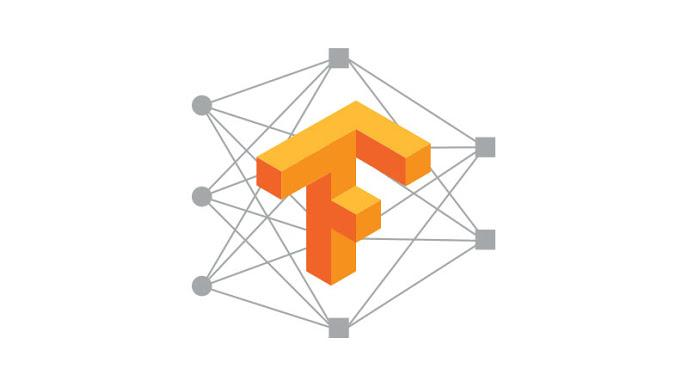Dataset API是TensorFlow 1.3版本中引入的一个新的模块，主要服务于数据读取，构建输入数据的pipeline。

## Dataset API的导入

``tf.contrib.data.Dataset``

``tf.data.Dataset``

## 基本概念：Dataset与Iterator

Dataset可以看作是相同类型“元素”的有序列表。在实际使用时，单个“元素”可以是向量，也可以是字符串、图片，甚至是tuple或者dict。

``````import tensorflow as tf
import numpy as np

dataset = tf.data.Dataset.from_tensor_slices(np.array([1.0, 2.0, 3.0, 4.0, 5.0]))``````

``````iterator = dataset.make_one_shot_iterator()
one_element = iterator.get_next()
with tf.Session() as sess:
for i in range(5):
print(sess.run(one_element))``````

``````dataset = tf.data.Dataset.from_tensor_slices(np.array([1.0, 2.0, 3.0, 4.0, 5.0]))
iterator = dataset.make_one_shot_iterator()
one_element = iterator.get_next()
with tf.Session() as sess:
try:
while True:
print(sess.run(one_element))
except tf.errors.OutOfRangeError:
print("end!")``````

``````import tensorflow.contrib.eager as tfe
tfe.enable_eager_execution()

dataset = tf.data.Dataset.from_tensor_slices(np.array([1.0, 2.0, 3.0, 4.0, 5.0]))

for one_element in tfe.Iterator(dataset):
print(one_element)``````

## 从内存中创建更复杂的Dataset

``dataset = tf.data.Dataset.from_tensor_slices(np.array([1.0, 2.0, 3.0, 4.0, 5.0]))``

``dataset = tf.data.Dataset.from_tensor_slices(np.random.uniform(size=(5, 2)))``

tf.data.Dataset.from_tensor_slices同样支持创建这种dataset，例如我们可以让每一个元素是一个词典：

``````dataset = tf.data.Dataset.from_tensor_slices(
{
"a": np.array([1.0, 2.0, 3.0, 4.0, 5.0]),
"b": np.random.uniform(size=(5, 2))
}
)``````

``````dataset = tf.data.Dataset.from_tensor_slices(
(np.array([1.0, 2.0, 3.0, 4.0, 5.0]), np.random.uniform(size=(5, 2)))
)``````

## 对Dataset中的元素做变换：Transformation

Dataset支持一类特殊的操作：Transformation。一个Dataset通过Transformation变成一个新的Dataset。通常我们可以通过Transformation完成数据变换，打乱，组成batch，生成epoch等一系列操作。

• map
• batch
• shuffle
• repeat

（1）map

map接收一个函数，Dataset中的每个元素都会被当作这个函数的输入，并将函数返回值作为新的Dataset，如我们可以对dataset中每个元素的值加1：

``````dataset = tf.data.Dataset.from_tensor_slices(np.array([1.0, 2.0, 3.0, 4.0, 5.0]))
dataset = dataset.map(lambda x: x + 1) # 2.0, 3.0, 4.0, 5.0, 6.0``````

（2）batch

batch就是将多个元素组合成batch，如下面的程序将dataset中的每个元素组成了大小为32的batch：

``dataset = dataset.batch(32)``

（3）shuffle

shuffle的功能为打乱dataset中的元素，它有一个参数buffersize，表示打乱时使用的buffer的大小：

``dataset = dataset.shuffle(buffer_size=10000)``

（4）repeat

repeat的功能就是将整个序列重复多次，主要用来处理机器学习中的epoch，假设原先的数据是一个epoch，使用repeat(5)就可以将之变成5个epoch：

``dataset = dataset.repeat(5)``

``dataset = dataset.repeat()``

## 例子：读入磁盘图片与对应label

``````# 函数的功能时将filename对应的图片文件读进来，并缩放到统一的大小
def _parse_function(filename, label):
image_decoded = tf.image.decode_image(image_string)
image_resized = tf.image.resize_images(image_decoded, [28, 28])
return image_resized, label

# 图片文件的列表
filenames = tf.constant(["/var/data/image1.jpg", "/var/data/image2.jpg", ...])
# label[i]就是图片filenames[i]的label
labels = tf.constant([0, 37, ...])

# 此时dataset中的一个元素是(filename, label)
dataset = tf.data.Dataset.from_tensor_slices((filenames, labels))

# 此时dataset中的一个元素是(image_resized, label)
dataset = dataset.map(_parse_function)

# 此时dataset中的一个元素是(image_resized_batch, label_batch)
dataset = dataset.shuffle(buffersize=1000).batch(32).repeat(10)``````

• 运行dataset = tf.data.Dataset.from_tensor_slices((filenames, labels))后，dataset的一个元素是(filename, label)。filename是图片的文件名，label是图片对应的标签。
• 之后通过map，将filename对应的图片读入，并缩放为28x28的大小。此时dataset中的一个元素是(image_resized, label)
• 最后，dataset.shuffle(buffersize=1000).batch(32).repeat(10)的功能是：在每个epoch内将图片打乱组成大小为32的batch，并重复10次。最终，dataset中的一个元素是(image_resized_batch, label_batch)，image_resized_batch的形状为(32, 28, 28, 3)，而label_batch的形状为(32, )，接下来我们就可以用这两个Tensor来建立模型了。

## Dataset的其它创建方法....

• tf.data.TextLineDataset()：这个函数的输入是一个文件的列表，输出是一个dataset。dataset中的每一个元素就对应了文件中的一行。可以使用这个函数来读入CSV文件。
• tf.data.FixedLengthRecordDataset()：这个函数的输入是一个文件的列表和一个record_bytes，之后dataset的每一个元素就是文件中固定字节数record_bytes的内容。通常用来读取以二进制形式保存的文件，如CIFAR10数据集就是这种形式。
• tf.data.TFRecordDataset()：顾名思义，这个函数是用来读TFRecord文件的，dataset中的每一个元素就是一个TFExample。

## 更多类型的Iterator....

• initializable iterator
• reinitializable iterator
• feedable iterator

initializable iterator必须要在使用前通过sess.run()来初始化。使用initializable iterator，可以将placeholder代入Iterator中，这可以方便我们通过参数快速定义新的Iterator。一个简单的initializable iterator使用示例：

``````limit = tf.placeholder(dtype=tf.int32, shape=[])

dataset = tf.data.Dataset.from_tensor_slices(tf.range(start=0, limit=limit))

iterator = dataset.make_initializable_iterator()
next_element = iterator.get_next()

with tf.Session() as sess:
sess.run(iterator.initializer, feed_dict={limit: 10})
for i in range(10):
value = sess.run(next_element)
assert i == value``````

initializable iterator还有一个功能：读入较大的数组。

``````# 从硬盘中读入两个Numpy数组
features = data["features"]
labels = data["labels"]

features_placeholder = tf.placeholder(features.dtype, features.shape)
labels_placeholder = tf.placeholder(labels.dtype, labels.shape)

dataset = tf.data.Dataset.from_tensor_slices((features_placeholder, labels_placeholder))
iterator = dataset.make_initializable_iterator()
sess.run(iterator.initializer, feed_dict={features_placeholder: features,
labels_placeholder: labels})``````

reinitializable iterator和feedable iterator相比initializable iterator更复杂，也更加少用，如果想要了解它们的功能，可以参阅官方介绍，这里就不再赘述了。